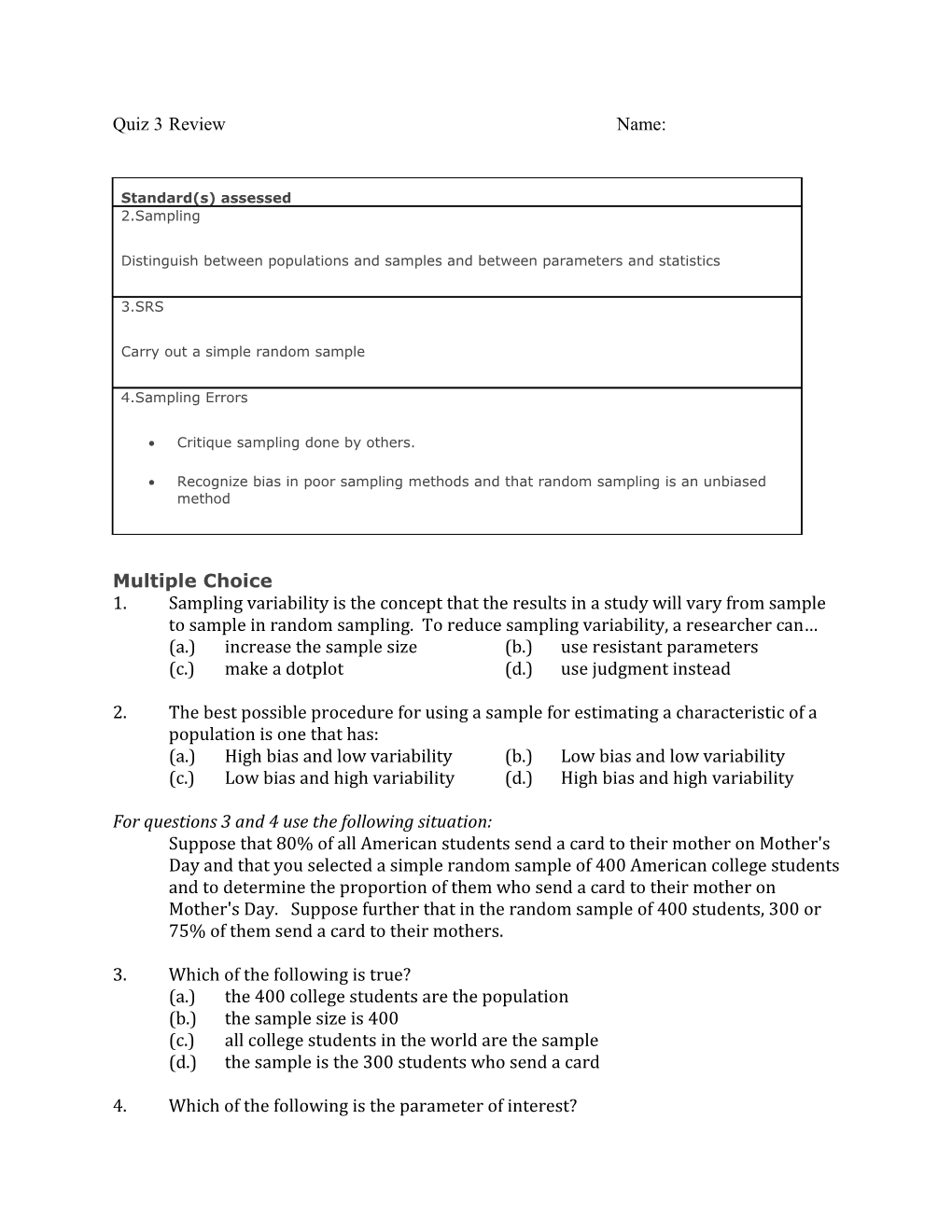# (A.) Increase the Sample Size(B.)Use Resistant ParametersQuiz 3ReviewName:

Standard(s) assessed
2.Sampling
Distinguish between populations and samples and between parameters and statistics
3.SRS
Carry out a simple random sample
4.Sampling Errors
• Critique sampling done by others.
• Recognize bias in poor sampling methods and that random sampling is an unbiased method

Multiple Choice

1.Sampling variability is the concept that the results in a study will vary from sample to sample in random sampling. To reduce sampling variability, a researcher can…

(a.) increase the sample size(b.)use resistant parameters

2. The best possible procedure for using a sample for estimating a characteristic of a population is one that has:

(a.)High bias and low variability(b.)Low bias and low variability

(c.)Low bias and high variability(d.)High bias and high variability

For questions 3 and 4 use the following situation:

Suppose that 80% of all American students send a card to their mother on Mother's Day and that you selected a simple random sample of 400 American college students and to determine the proportion of them who send a card to their mother on Mother's Day. Suppose further that in the random sample of 400 students, 300 or 75% of them send a card to their mothers.

3.Which of the following is true?

(a.)the 400 college students are the population

(b.)the sample size is 400

(c.)all college students in the world are the sample

(d.)the sample is the 300 students who send a card

4.Which of the following is the parameter of interest?

(a.)the proportion of college students who send a card on Mother’s Day

(b.)the proportion of the 400 students in the sample who send a card

(c.)the proportion of all college students in the world who send a card

(d.)the 300 students in the sample who sent a card

5.Suppose we are interested in the average reading achievement test

score of the currently enrolled students in Edison Elementary School.

The tests would be the observational units. The test scores would be ______while the average score of all students in one teacher’s class is ______.

(a.) an observational unit, a sample

(b.) a sample, a parameter

(c.)a variable, a sample

(d.) a population, a variable

6.A sample is:

(a.) a number resulting from the manipulation of raw data according to specified rules.

(b.) a subset of a population.

(c.) a characteristic of a population which is measurable.

(d.) a complete set of individuals, objects, or measurements having some common observable characteristic.

7. Characteristics of a sample are called ______, while those of a

population are termed ______.

(a.) statistics; measures

(b.) parameters; statistics

(c.) statistics; variables

(d.) statistics; parameters

8.Which of the following is really a random sample?

(a.) The teacher wants to show a representative sample of student work, so she picks one good paper, one average paper, and one bad paper.

(b.) The gym teacher asks the students to line up and count off by four’s. All the “one’s” are selected to be one team.

(c.) The name of each person in the population is put into a hat and mixed well. Names are drawn from the hat without looking.

(d.) The surveyor picks a sample of people he feels is representative of the population.

9.A simple random sample is one where

a) you use your best judgment to find a representative group.

b) you choose each item without really thinking about it.

c) you select a group that shares some characteristic.

d) all of the above are true.

e) none of the above are true.

10.The generation of American children born in the 1990s and 2000s have been dubbed “Generation M” because of the impact of media exposure. To study the television viewing habits of Generation M teenagers, researchers conducted a survey of 200 teenagers attending a MTV spring break event.

Describe each of the following:

Population:

Sample:

Parameter:

Statistic:

Do you think the sampling method used is biased? Explain.

11. Select an SRS (Simple Random Sample) of 5 students using the numbered list below and the table of random digits provided. Start at the beginning of the secondline to randomly select two-digit numbers corresponding to student names.

Random number table:

40603161528323537361987832483839793809547686532713

40941535856995860916710189056184505539806473585140

73505834725595317957114462261834771257772706413526

39412160131144289320113074939639805122495765688686

57994767485462748511786463328735524545220879556273

Sample selected: ______

______

______

______

______

1 / Sofia
2 / Eassa
3 / Jeffrey
4 / Shakoya
5 / John
6 / Rebecca
7 / William
8 / Johanna
9 / Allyson
10 / Brandon
11 / Dara
12 / Jay
13 / Nicole
14 / Francis
15 / Audrey
16 / Anthoula
17 / Hiep
18 / Sean
19 / Shanira
20 / Alexis
21 / Melanie
22 / Ian
23 / Rebecca